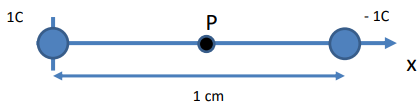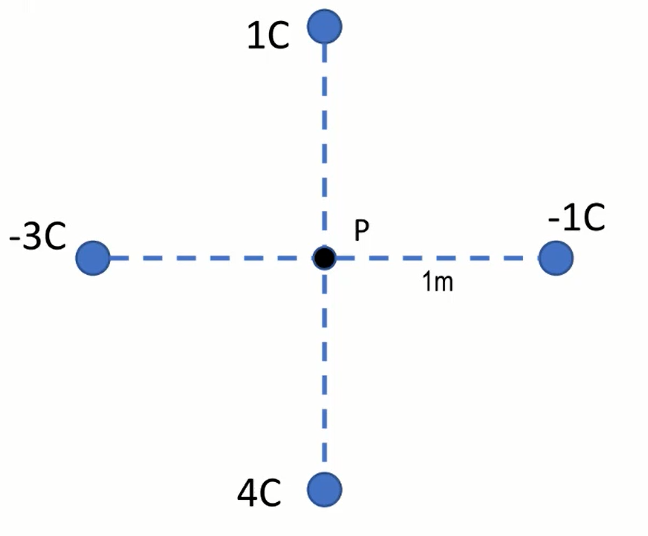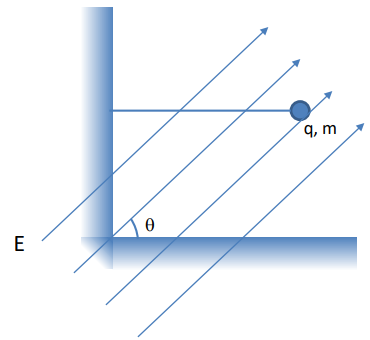Clutch Prep is now a part of Pearson
Ch 20: Electric Fields and ForcesWorksheetSee all chapters

# Electric Field

See all sections
Sections
Electric Charge
Charging Objects
Charging By Induction
Conservation of Charge
Dipole Moment
Coulomb's Law (Electric Force)
Electric Field
Electric Fields in Capacitors
Electric Field Lines
Electric Fields in Conductors

Concept #1: Intro to Electric Fields

Practice: A 1.5μC charge, with a mass of 50g, is in the presence of an electric field that perfectly balances its gravity. What magnitude does the electric field need to be, and in what direction does it need to point?

Concept #2: Electric Field due to a Point Charge

Example #1: Zero Electric Field due to Two Charges

Practice: If two equal charges are separated by some distance, they form an electric dipole. Find the electric field at the center of an electric dipole, given by the point P in the following figure, formed by a 1C and a −1C charge separated by 1 cm.Example #2: Electric Field Above Two Charges (Triangle)

Practice: 4 charges are arranged as shown in the following figure. Find the magnitude of the electric field at the center of the arrangement, indicated by the point P.Example #3: Balancing a Pendulum in Electric Field

Practice: In the following figure, a mass m is balanced such that its tether is perfectly horizontal. If the mass is m and the angle of the electric field is 𝜃, what is the magnitude of the electric field, E?